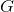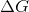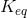GRE Subject Test: Biochemistry, Cell, and Molecular Biology : Help with Thermodynamics and Energetics

Example Questions

Example Question #1 : Biochemistry

Which of the following statements is not true regarding Gibbs free energy,If theof a reaction is positive, the reaction is not favorable and likely requires external energy to proceed

If theof a reaction is less than zero, the reaction is endergonic

If theof a reaction is zero, the reaction is at equilibrium

Theof a reaction is partially dependent on the temperature of the system

Theof a reaction is partially dependent on enthalpy of the system

If theof a reaction is less than zero, the reaction is endergonic

Explanation:

If theof a reaction is less than zero, the reaction will in fact be exergonic. These reactions will be favorable and spontaneous, and energy is released in these reactions. Thus, endergonic is incorrect.

Example Question #2 : Biochemistry

The equilibrium constantof any given chemical reaction is __________ on the temperature and pressure of the reactants and products and is __________ of the reaction rate.

dependent . . . one-half

Independent . . . one-half

independent . . . dependent

dependent . . . dependent

dependent . . . independent

dependent . . . independent

Explanation:

The temperature and pressure of the reactants and products of any given reaction will determine the value of. However, this is entirely dependent of the reaction rate, which is determined by how the concentrations of the reactants and products change over the course of the reaction. Additionally, every reaction is unique, so "one-half" or any other exact metric cannot be applied as the definition.

Example Question #3 : Biochemistry

Which of the following best describes a reaction that has reached equilibrium?is greater than zero, and the forward and reverse reactions are occurring at equal rates.is zero, and the forward reaction is occurring spontaneously.is less than zero, and the forward and reverse reactions are occurring at equal rates.is zero, and the forward and reverse reactions are occurring at equal rates.is zero, and both the forward and reverse reactions have stopped occurring completely.is zero, and the forward and reverse reactions are occurring at equal rates.

Explanation:

In equilibrium, both the forward and reverse reactions continue to occur, but they do so in a way that is equal and thus, there is no net change of reactants and products in the system.is zero in this case because net changes to the system have ceased, and thus the free energy is no longer in flux.

Example Question #4 : Biochemistry

__________ reactions have athat is less than zero, and thus they occur spontaneously. This is because the products in these reactions have lower bond energies than the reactants, and when that energy is released it is converted to __________.

exothermic . . . electricity

endothermic . . . heat

exothermic . . . light

exothermic . . . heat

endothermic . . . light

Exothermic reactions release heat (think exothermic = explosion = creates heat), and this is due to the release of energy from the reactant bonds. These reactions haveless than zero. Endothermic reactions are just the opposite and actually require energy to occur, and therefore do not occur spontaneously nor release heat.